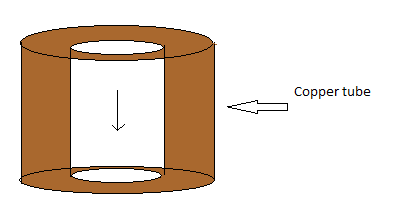# Magnet falling in a Copper tubeIf you have already seen this then it's okay, if not then see this.

Copper pipe and magnet falling in it

I recently saw this video and got interested in analysing the situation and I took a simplistic situation.

Assume a infinitely long copper tube which is in the shape of a right uniform hollow cylinder with inside radius ${r}_{1}$, outer radius ${r}_{2}$.

A magnet of magnetic moment $M$ is dropped from a point on the axis of the cylinder with the magnetic moment vector( or you may interpret it as north south line) being along the axis of the cylinder.

If the terminal velocity of the magnet is given by :

$\displaystyle {v}_{T} = \frac { { a }^{ b }{ m }^{ c }{ g }^{ d }{ \rho }^{ e } }{ f{ M }^{ h }{ \mu }_{ 0 }^{ i } }\left (\frac { 1 }{ { r }_{ 1 }^{ -j }-{ r }_{ 2 }^{ -j } }\right )$

Find $a+b+c+d+e+f+h+i+j$.

Details and assumptions:

1) $m$= Mass of magnet, $g$= Acceleration due to gravity, $M$ = Magnetic moment ,

$\rho$=Resistivity of copper(you can also call it specific resistance), ${\mu}_{0}$ = Permeability of vacuum.

2) $a,b,c,d,e,f,g,h,i$ are positive integers all less than $20$ where $a$ is a prime integer, $a$,$f$ are co- prime integers.

3) Assume the magnet to be a point dipole with magnetic moment $M$ and pointing along the axis of the cylinder as shown in the figure.

×